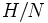# Finite index implies powering-invariant

This article gives the statement and possibly, proof, of an implication relation between two subgroup properties. That is, it states that every subgroup satisfying the first subgroup property (i.e., subgroup of finite index) must also satisfy the second subgroup property (i.e., powering-invariant subgroup)
View all subgroup property implications | View all subgroup property non-implications
Get more facts about subgroup of finite index|Get more facts about powering-invariant subgroup

## Statement

Suppose$G$ is a group and$H$ is a subgroup of finite index in$G$. Then,$H$ is a powering-invariant subgroup of$G$. In other words, if$p$ is a prime number such that$G$ is powered over$p$, then$H$ is also powered over$p$.

## Proof

Given: A group$G$, a subgroup$H$ of finite index in$G$.

To prove:$H$ is powering-invariant in$G$.

Proof:

Step no. Assertion/construction Facts used Given data used Previous steps used Explanation
1 Let$N$ be the normal core of$H$ in$G$. Then,$N$ is a normal subgroup of finite index in$G$. Fact (1)$H$ has finite index in$G$. Fact-given direct.
2$N$ is a quotient-powering-invariant of$G$. Fact (2) Step (1) Step-fact direct.
3$H/N$ is a powering-invariant subgroup of$G/N$. Fact (3) Step (1) By Step (1),$G/N$ is finite, hence$H/N$, as a subgroup of it, is finite. Thus, by Fact (3), it is powering-invariant in$G$.
4$H$ is powering-invariant in$G$. Fact (4) Steps (2) and (3) Step-combination direct.